Courses

# Influence Lines for Beams - 1 Civil Engineering (CE) Notes | EduRev

## Civil Engineering (CE) : Influence Lines for Beams - 1 Civil Engineering (CE) Notes | EduRev

The document Influence Lines for Beams - 1 Civil Engineering (CE) Notes | EduRev is a part of the Civil Engineering (CE) Course Structural Analysis.
All you need of Civil Engineering (CE) at this link: Civil Engineering (CE)

Instructional Objectives:

The objectives of this lesson are as follows:

• How to draw qualitative influence lines?
• Understand the behaviour of the beam under rolling loads
• Construction of influence line when the beam is loaded with uniformly distributed load having shorter or longer length than the span of the beam.

Müller Breslau Principle for Qualitative Influence Lines

In 1886, Heinrich Müller Breslau proposed a technique to draw influence lines quickly. The Müller Breslau Principle states that the ordinate value of an influence line for any function on any structure is proportional to the ordinates of the deflected shape that is obtained by removing the restraint corresponding to the function from the structure and introducing a force that causes a unit displacement in the positive direction.

Let us say, our objective is to obtain the influence line for the support reaction at A for the beam shown in Figure 38.1.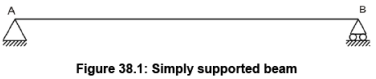First of all remove the support corresponding to the reaction and apply a force (Figure 38.2) in the positive direction that will cause a unit displacement in the direction of RA. The resulting deflected shape will be proportional to the true influence line (Figure 38.3) for the support reaction at A.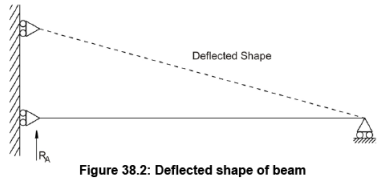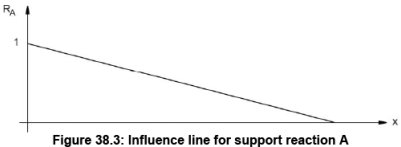The deflected shape due to a unit displacement at A is shown in Figure 38.2 and matches with the actual influence line shape as shown in Figure 38.3. Note that the deflected shape is linear, i.e., the beam rotates as a rigid body without any curvature. This is true only for statically determinate systems.

Similarly some other examples are given below.

Here we are interested to draw the qualitative influence line for shear at section C of overhang beam as shown in Figure 38.4.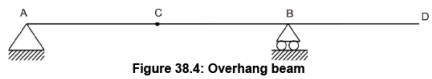As discussed earlier, introduce a roller at section C so that it gives freedom to the beam in vertical direction as shown in Figure 38.5.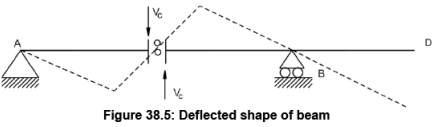Now apply a force in the positive direction that will cause a unit displacement in the direction of VC. The resultant deflected shape is shown in Figure 38.5. Again, note that the deflected shape is linear. Figure 38.6 shows the actual influence, which matches with the qualitative influence.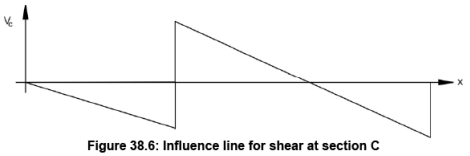In this second example, we are interested to draw a qualitative influence line for moment at C for the beam as shown in Figure 38.7.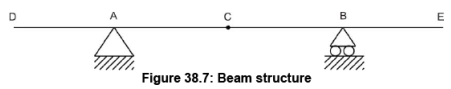In this example, being our objective to construct influence line for moment, we will introduce hinge at C and that will only permit rotation at C. Now apply moment in the positive direction that will cause a unit rotation in the direction of Mc. The deflected shape due to a unit rotation at C is shown in Figure 38.8 and matches with the actual shape of the influence line as shown in Figure 38.9.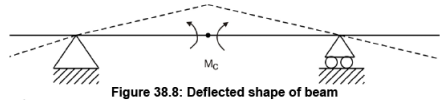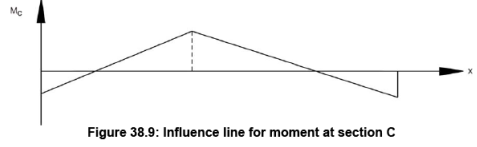Maximum shear in beam supporting UDLs

If UDL is rolling on the beam from one end to other end then there are two possibilities. Either Uniformly distributed load is longer than the span or uniformly distributed load is shorter than the span. Depending upon the length of the load and span, the maximum shear in beam supporting UDL will change. Following section will discuss about these two cases. It should be noted that for maximum values of shear, maximum areas should be loaded.

UDL longer than the span

Let us assume that the simply supported beam as shown in Figure 38.10 is loaded with UDL of w moving from left to right where the length of the load is longer than the span. The influence lines for reactions RA, RB and shear at section C located at x from support A will be as shown in Figure 38.11, 38.12 and 38.13 respectively. UDL of intensity w per unit for the shear at supports A and B will be given by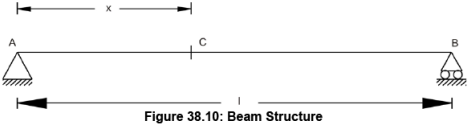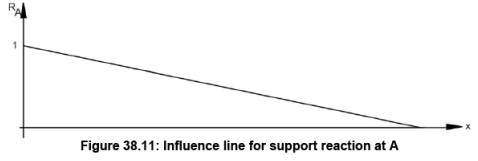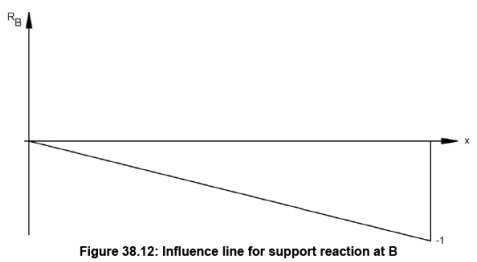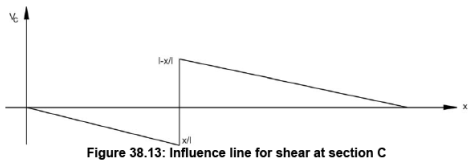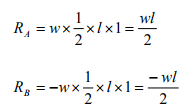Suppose we are interested to know shear at given section at C. As shown in Figure 38.13, maximum negative shear can be achieved when the head of the load is at the section C. And maximum positive shear can be obtained when the tail of the load is at the section C. As discussed earlier the shear force is computed by intensity of the load multiplied by the area of influence line diagram covered by load. Hence, maximum negative shear is given by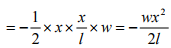and maximum positive shear is given by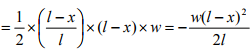UDL shorter than the span

When the length of UDL is shorter than the span, then as discussed earlier, maximum negative shear can be achieved when the head of the load is at the section. And maximum positive shear can be obtained when the tail of the load is at the section.  As discussed earlier the shear force is computed by the load intensity multiplied by the area of influence line diagram covered by load. The example is demonstrated in previous lesson.

Maximum bending moment at sections in beams supporting UDLs.

Like the previous section discussion, the maximum moment at sections in beam supporting UDLs can either be due to UDL longer than the span or due to ULD shorter than the span. Following paragraph will explain about computation of moment in these two cases.

UDL longer than the span

Let us assume the UDL longer than the span is traveling from left end to right hand for the beam as shown in Figure 38.14. We are interested to know maximum moment at C located at x from the support A. As discussed earlier, the maximum bending moment is given by the load intensity multiplied by the area of influence line (Figure 38.15) covered. In the present case the load will cover the completed span and hence the moment at section C can be given by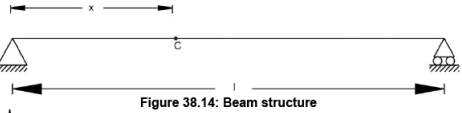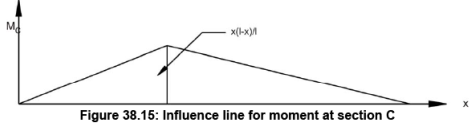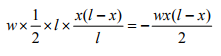Suppose the section C is at mid span, then maximum moment is given by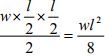UDL shorter than the span

As shown in Figure 38.16, let us assume that the UDL length y is smaller than the span of the beam AB. We are interested to find maximum bending moment at section C located at x from support A. Let say that the mid point of UDL is located at D as shown in Figure 38.16 at distance of z from support A. Take moment with reference to A and it will be zero.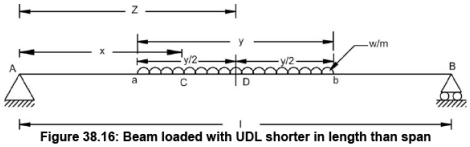Hence, the reaction at B is given by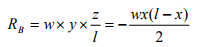And moment at C will be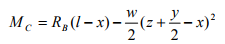Substituting value of reaction B in above equation, we can obtain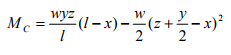To compute maximum value of moment at C, we need to differentiate above given equation with reference to z and equal to zero.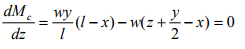Therefore,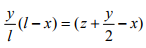Using geometric expression, we can state that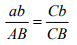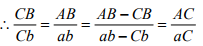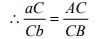The expression states that for the UDL shorter than span, the load should be placed in a way so that the section divides it in the same proportion as it divides the span. In that case, the moment calculated at the section will give maximum moment value.

Offer running on EduRev: Apply code STAYHOME200 to get INR 200 off on our premium plan EduRev Infinity!

## Structural Analysis

30 videos|122 docs|28 tests

,

,

,

,

,

,

,

,

,

,

,

,

,

,

,

,

,

,

,

,

,

;